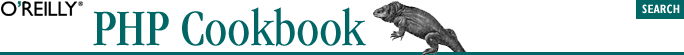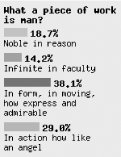home | O'Reilly's CD bookshelfs | FreeBSD | Linux | Cisco | Cisco Exam## 15.10. Program: Generating Bar Charts from Poll Results

When displaying the results of a poll, it can be more effective to generate a colorful bar chart instead of just printing the results as text. The function shown in Example 15-4 uses GD to create an image that displays the cumulative responses to a poll question.

#### Example 15-4. Graphical bar charts

```function pc_bar_chart(\$question, \$answers) {

// define colors to draw the bars
\$colors = array(array(255,102,0), array(0,153,0),
array(51,51,204), array(255,0,51),
array(255,255,0), array(102,255,255),
array(153,0,204));

// define some spacing values and other magic numbers
\$line_width = 20;
\$scale = \$line_width * 7.5;
\$bar_height = 10;

\$x = \$y = \$padding;

// allocate a large palette for drawing, since we don't know
// the image length ahead of time
\$image = ImageCreate(150, 500);
\$bg_color = ImageColorAllocate(\$image, 224, 224, 224);
\$black = ImageColorAllocate(\$image, 0, 0, 0);

// print the question
\$wrapped = explode("\n", wordwrap(\$question, \$line_width));
foreach (\$wrapped as \$line) {
ImageString(\$image, 3, \$x, \$y , \$line, \$black);
\$y += 12;
}

// print the answers
for (\$i = 0; \$i < count(\$answers['answer']); \$i++) {

// format percentage

// grab color
\$c = \$i % count(\$colors); // handle cases with more bars than colors
\$text_color = ImageColorAllocate(\$image, \$colors[\$c],
\$colors[\$c], \$colors[\$c]);

// draw bar and percentage numbers
ImageFilledRectangle(\$image, \$x, \$y, \$x + \$bar,
\$y + \$bar_height, \$text_color);
ImageString(\$image, 3, \$x + \$bar + \$padding, \$y,
"\$percent%", \$black);

\$y += 12;

foreach (\$wrapped as \$line) {
ImageString(\$image, 2, \$x, \$y, \$line, \$black);
\$y += 12;
}

\$y += 7;
}

// crop image by copying it
\$chart = ImageCreate(150, \$y);
ImageCopy(\$chart, \$image, 0, 0, 0, 0, 150, \$y);

// deliver image
ImagePNG(\$chart);

// clean up
ImageDestroy(\$image);
ImageDestroy(\$chart);
}```
```// Act II. Scene II.
\$question = 'What a piece of work is man?';

\$answers['answer'][  ] = 'In form, in moving, how express and admirable';

\$answers['answer'][  ] = 'In action how like an angel';